# Viète theorem

on roots

A theorem which establishes relations between the roots and the coefficients of a polynomial. Letbe a polynomial of degreewith coefficients from some field and with leading coefficient 1. The polynomialsplits over a field containing all the roots of(e.g. over the splitting field of, cf. Splitting field of a polynomial) into linear factors:where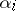are the roots of,. Viète's theorem asserts that the following relations (Viète's formulas) hold: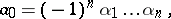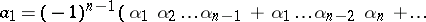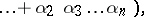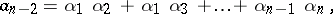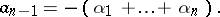F. Viète  proved this relation for all, but for positive roots only; the general form of Viète's theorem was established by A. Girard .

How to Cite This Entry:
Viète theorem. Encyclopedia of Mathematics. URL: http://encyclopediaofmath.org/index.php?title=Vi%C3%A8te_theorem&oldid=14535
This article was adapted from an original article by V.N. Remeslennikov (originator), which appeared in Encyclopedia of Mathematics - ISBN 1402006098. See original article